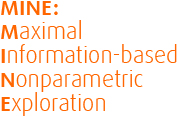# Overview## Approaching a new dataset

One way of beginning to explore a many-dimensional dataset is to calculate some measure of dependence for each pair of variables, rank the pairs by their scores, and examine the top-scoring pairs. For this strategy to work, the statistic used to measure dependence should have the following two heuristic properties.

Generality: with sufficient sample size the statistic should capture a wide range of interesting associations, not limited to specific function types (such as linear, exponential, or periodic), or even to all functional relationships.

Equitability: the statistic should give similar scores to equally noisy relationships of different types. For instance, a linear relationship with an R2 of 0.80 should receive approximately the same score as a sinusoidal relationship with an R2 of 0.80.

## Exploring data with MIC

The maximal information coefficient (MIC) is a measure of bivariate dependence developed with the goals of generality and equitability. The original paper describing MIC shows that it comes very close to achieving both goals simultaneously, and that it outperforms other methods in this regard.

## Other MINE statistics

A variety of related statistics arise naturally as side products of the calculation of MIC. These statistics can be used to characterize the relationships identified by MIC. They include measures of monotonicity, non-linearity, closeness to being a function, and complexity of relationships.